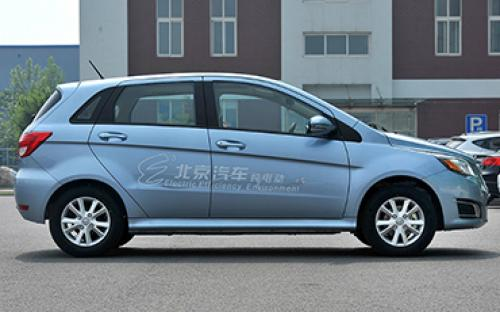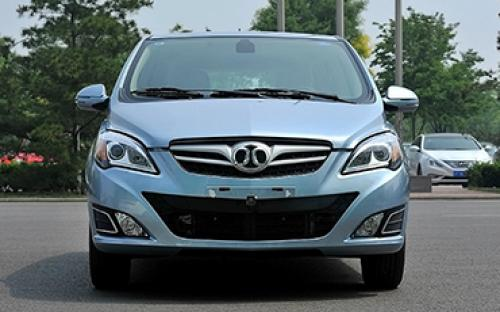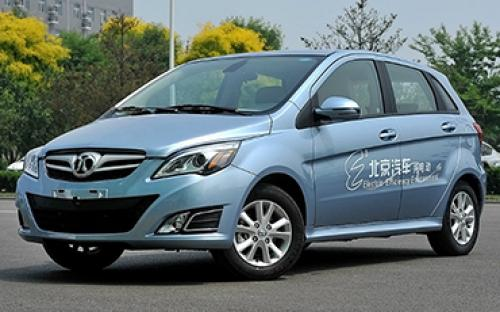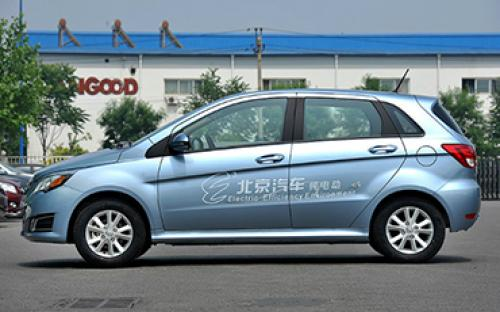### 北汽新能源 E150EV6 种颜色可选2014款最低售价：22.08 万元起

3998(mm)1720(mm)1503(mm)##### 配置亮点：
• 胎压监测装置

• ISOFIX儿童座椅接口

• 车身稳定控制(ESC/ESP/DSC等)

• 电动天窗

• 定速巡航

• 后倒车雷达

• 真皮座椅

• GPS导航系统

• 氙气大灯

• 后视镜加热

• 提交

北汽新能源 E150EV 绕车实拍• 北汽新能源 E150EV 在售车型

排量 车型 厂商指导价 本地最低报价 购车工具
-L
两厢 E150 EV 电动时尚版 纯电动 电动车单速变速箱
22.08万
两厢 E150 EV 电动科技版 纯电动 电动车单速变速箱
23.08万

北汽新能源 E150EV 经销商

查看更多 >>

### 北汽新能源 E150EV 动力加速

E150EV 0-100公里加速时间分布在 0.0-秒 属于 超跑级

动力级别 加速时间 车型

北汽新能源 E150EV 视频

北汽新能源 E150EV 新闻资讯

# “车辆工程”这门专业不错

新闻中心 超过5223次关注

虽然只是一名刚入职不久的小专员，但在我们科室只有我是名牌大学汽车专业毕业，所以平时在单位谈论起买车话题时多少有些“傲娇”，总是想表现出自己的“专业性”。...

# 节能环保新选择 能源车型推荐

导购 超过3705次关注

北汽E150EV是基于E系列所打造的一款纯电动车型，该车完全沿用了汽油版车型的设计，造型酷似老款的奔驰B级。目前北汽E150EV已面向个人销售，厂家指导价为25.98万，北...

# 距离成熟还很远 测试北汽E150 EV电动车

评测 超过3446次关注

北京市小客车新能源指标首次摇号进行，3094名个人/单位中签；同日，北京首批新能源汽车生产企业和产品目录正式公布，北汽E150EV、比亚迪e6电动车入围北京新能源车第...

# 北汽新能源启动“卫•蓝先锋”公益活动

文化 超过4135次关注

由北京新能源汽车股份有限公司主办的“卫·蓝先锋”启动仪式于6月5日举行，此活动目的是鼓励北京市民绿色出行，同时主办方还聘请了四位知名人士成为绿色出行公益大...

# 绅宝EV将于7月上市 北汽新能源规划曝光

新闻 超过3501次关注

日前，北汽新能源汽车股份有限公司副总经理、营销公司总经理张勇，北汽集团副总工程师林逸接受了汽车之家的专访，并对未来北汽新能源的产品规划以及销售网络布局等...

# 北汽E150 EV北京上市 售22.08-23.08万

新闻 超过3347次关注

北京首批新能源汽车生产企业和产品目录正式公布，北汽电动车E150EV车出现在了目录中。日前，北汽正式公布了E150EV的售价。此次上市的E150EV共有时尚版和科技版两款...

# 北京新能源车补贴目录发布 两款车入围

新闻 超过3842次关注

北京经信委公布了第一批第一期《北京市示范应用新能源小客车生产企业及产品目录》（下文简称《目录》），北汽E150EV、比亚迪e6电动车入围。

猜你喜欢

﻿
• 快速找车
• 选择品牌
• 选择品牌
• A  奥迪
• A  阿斯顿·马丁
• A  阿尔法·罗密欧
• B  宝沃
• B  布加迪
• B  巴博斯
• B  保时捷
• B  宾利
• B  奔驰
• B  宝马
• B  本田
• B  别克
• B  标致
• B  比亚迪
• B  宝骏
• B  北汽制造
• B  北汽新能源
• B  北汽幻速
• B  北汽威旺
• B  北京汽车
• B  奔腾
• B  北汽绅宝
• C  长安
• C  长安商用
• C  长城
• C  昌河
• D  大众
• D  道奇
• D  DS
• D  东南
• D  东风风神
• D  东风风行
• D  东风小康
• D  东风风度
• D  东风
• F  福特
• F  丰田
• F  菲亚特
• F  法拉利
• F  福田
• F  福迪
• F  福汽启腾
• G  观致
• G  广汽传祺
• G  广汽吉奥
• G  GMC
• H  红旗
• H  汉腾汽车
• H  哈弗
• H  哈飞
• H  海格
• H  海马
• H  华颂
• H  黄海
• H  华泰
• H  恒天
• J  吉利汽车
• J  捷豹
• J  Jeep
• J  江淮
• J  江铃
• J  金杯
• J  九龙
• J  金旅
• K  凯翼
• K  凯迪拉克
• K  克莱斯勒
• K  科尼塞克
• K  卡威
• K  开瑞
• L  路虎
• L  林肯
• L  劳斯莱斯
• L  兰博基尼
• L  雷克萨斯
• L  铃木
• L  雷诺
• L  理念
• L  力帆
• L  莲花汽车
• L  猎豹
• L  路特斯
• L  陆风
• M  马自达
• M  MG
• M  MINI
• M  玛莎拉蒂
• M  摩根
• M  迈凯轮
• N  纳智捷
• O  欧宝
• O  讴歌
• O  欧朗
• Q  奇瑞
• Q  起亚
• Q  启辰
• R  日产
• R  荣威
• R  瑞麒
• S  三菱
• S  斯威汽车
• S  萨博
• S  smart
• S  斯柯达
• S  斯巴鲁
• S  思铭
• S  双龙
• S  上汽大通
• S  双环
• T  特斯拉
• T  腾势
• W  沃尔沃
• W  五菱汽车
• W  五十铃
• W  威兹曼
• W  威麟
• X  现代
• X  雪佛兰
• X  雪铁龙
• X  西雅特
• Y  一汽
• Y  英菲尼迪
• Y  英致
• Y  依维柯
• Y  野马汽车
• Y  永源
• Z  众泰
• Z  中华
• Z  中兴
• Z  知豆
• 选择车系
• 选择车系
• 车型对比
• 选择品牌
• 选择品牌
• A  奥迪
• A  阿斯顿·马丁
• A  阿尔法·罗密欧
• B  宝沃
• B  布加迪
• B  巴博斯
• B  保时捷
• B  宾利
• B  奔驰
• B  宝马
• B  本田
• B  别克
• B  标致
• B  比亚迪
• B  宝骏
• B  北汽制造
• B  北汽新能源
• B  北汽幻速
• B  北汽威旺
• B  北京汽车
• B  奔腾
• B  北汽绅宝
• C  长安
• C  长安商用
• C  长城
• C  昌河
• D  大众
• D  道奇
• D  DS
• D  东南
• D  东风风神
• D  东风风行
• D  东风小康
• D  东风风度
• D  东风
• F  福特
• F  丰田
• F  菲亚特
• F  法拉利
• F  福田
• F  福迪
• F  福汽启腾
• G  观致
• G  广汽传祺
• G  广汽吉奥
• G  GMC
• H  红旗
• H  汉腾汽车
• H  哈弗
• H  哈飞
• H  海格
• H  海马
• H  华颂
• H  黄海
• H  华泰
• H  恒天
• J  吉利汽车
• J  捷豹
• J  Jeep
• J  江淮
• J  江铃
• J  金杯
• J  九龙
• J  金旅
• K  凯翼
• K  凯迪拉克
• K  克莱斯勒
• K  科尼塞克
• K  卡威
• K  开瑞
• L  路虎
• L  林肯
• L  劳斯莱斯
• L  兰博基尼
• L  雷克萨斯
• L  铃木
• L  雷诺
• L  理念
• L  力帆
• L  莲花汽车
• L  猎豹
• L  路特斯
• L  陆风
• M  马自达
• M  MG
• M  MINI
• M  玛莎拉蒂
• M  摩根
• M  迈凯轮
• N  纳智捷
• O  欧宝
• O  讴歌
• O  欧朗
• Q  奇瑞
• Q  起亚
• Q  启辰
• R  日产
• R  荣威
• R  瑞麒
• S  三菱
• S  斯威汽车
• S  萨博
• S  smart
• S  斯柯达
• S  斯巴鲁
• S  思铭
• S  双龙
• S  上汽大通
• S  双环
• T  特斯拉
• T  腾势
• W  沃尔沃
• W  五菱汽车
• W  五十铃
• W  威兹曼
• W  威麟
• X  现代
• X  雪佛兰
• X  雪铁龙
• X  西雅特
• Y  一汽
• Y  英菲尼迪
• Y  英致
• Y  依维柯
• Y  野马汽车
• Y  永源
• Z  众泰
• Z  中华
• Z  中兴
• Z  知豆
• 选择车系
• 选择车系
• 选择车型
• 选择车型
• 意见反馈